Terahertz multi-metal-wire hybrid-cladding hollow waveguide for refractive index sensing
College of Automation, Harbin Engineering University, Harbin 150001, China

† Corresponding author. E-mail: yuyingying58@hotmail.com

Project supported by the National Natural Science Foundation of China (Grant No. 51309059).

Abstract
Abstract

We propose a design of terahertz refractive index sensing based on the multi-metal-wire (MMW) hybrid-cladding hollow waveguide. The proposed terahertz hybrid-cladding hollow waveguide comprises one air core in the center surrounding MMW surrounded dielectric. The central air core is used for filling lossless measurands and transmitting terahertz light. In particular, the refractive index sensing is realized by measuring the mode field area (MFA) variation of radially polarized mode. The modal effective refractive index, mode field intensity distribution, and mode field area properties responding to the measurand refractive indexes for different operating frequencies and structure dimensions are investigated, respectively. Simulations show that the proposed terahertz refractive index sensor can realize easily the measurement of the measurand refractive index. Meanwhile, the effects of operating frequency and structure parameters on sensitivity and measurement accuracy are also studied. In view of the trade-off between sensitivity and measurement accuracy, the reasonable choice of the operating frequency and structure parameters can optimize appropriately the sensitivity and measurement accuracy, and the sensitivity can reach approximately 0.585 mm2/RIU (RIU is short for refraction index units) with the proper frequency and structure parameter.

1. Introduction

Terahertz (THz) radiation technologies have drawn increasing attention in recent years due to its unique properties compared with other wavelength bands, and significant potential applications in many areas such as sensing, communication, and imaging, etc. THz waveguide technology is also vital to the improvement of THz technology due to its potential ability to construct low loss and robust THz integrated systems. To date, THz waveguide composed of a variety of dielectrics/metals has been demonstrated. THz dielectric waveguides include dielectric ribbon waveguide, dielectric subwavelength fibers, and dielectric hollow core waveguide. However, because of high losses of dielectric materials in the terahertz range, metal-based THz waveguides have also been reported including metal wire waveguide, hollow metallic waveguide, and parallel plate metallic waveguide. Nevertheless, metal-based THz waveguide suffers bend and diffraction losses to some extent, which restricts the application of metal-based THz waveguide. Therefore, hybrid structure waveguide methods combining the merits of metal waveguide and dielectric waveguide have continued to develop over the past several years.

Recently, there has been an increasing interest in waveguide for parametric sensing (refractive index and strain sensing, etc.), due to its important applications in biochemistry and medicine. THz waveguide parametric sensor has also been extensively applied to bio-sensing filed because of the characteristics of low photonic energy and multiple frequency spectrum of THz radiation. Recently, a variety of THz waveguide parametric sensors were proposed and demonstrated, such as mechanically tunable metamaterial sensors, photonic crystal waveguide sensors, dielectric pipe waveguide sensors, and hybrid plasmonic waveguide refractive index sensors, which can realize effectively the measurement of the analyte refractive index.

In the present paper, a new type of THz waveguide refractive index sensor based on the multi-metal-wire hybrid-cladding hollow waveguide is proposed and demonstrated. Unlike the previously reported THz waveguide refractive index sensor, the proposed sensor detects the variation of mode field area in response to the changes of the measurand refractive index. For the different frequencies and structure dimensions of the proposed THz sensor, the variations of mode field area with measurand refractive index are investigated numerically. In addition, the variations of sensitivity and accuracy with frequency and geometric dimension of the device are also analyzed. The proposed THz waveguide refractive index sensor can be potentially applied to the detection of various gaseous measurands.

2. Structure design and theoretical analysis

The THz hybrid-cladding hollow waveguide considered here is a structure with multi-metal-wires inserted into the internal junction of the hollow dielectric waveguide. Figure 1 illustrates the transverse section of the proposed THz waveguide and the schematic diagram for refractive index sensor formed by the THz waveguide. As depicted in Fig. 1(a), eight metal wires are partly inserted into the dielectric cladding and partly exposed to the air core in the center. The air core is used for filling lossless measurands and transmitting terahertz light, and the dielectric cladding provides mechanical stability and isolation from the environment. In Fig. 1(a), d1 is the air core diameter, d2 is the metallic wire diameter, and D is the dielectric cladding diameter. Here, TOPAS and copper are chosen to be used for the dielectric cladding and metallic wires, respectively, for the reason that the TOPAS has a relatively low material absorption loss and copper is one of the best reflectors in the THz range and low-cost advantage.[28,29]

 Figure Option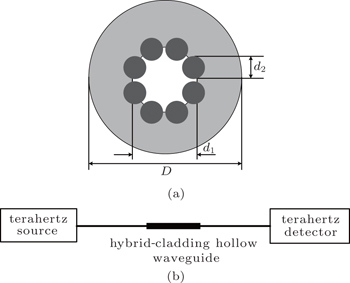Fig. 1. (a) Transverse section of the hybrid-cladding hollow waveguide, (b) schematic diagram of the set-up for THz refractive index sensor.
3. Results and discussion

Firstly, we comparatively study how our proposed multi-metal-wire hybrid-cladding hollow waveguide differs from a metallic inner coating (MIC) hollow dielectric waveguide. Here, the air core diameter d1, the metallic wire diameter d2, the dielectric cladding diameter D, and the operating frequency are assumed to be 1.60 mm, 0.49 mm, 4.00 mm, and 0.69 THz respectively. Note that the parameters of the proposed THz waveguide are fixed unless otherwise stated. We assume here that the MIC has geometry dimensions comparable to those of our proposed MMW. That is, the dielectric cladding diameter D = 4.00 mm, and the air core diameter d1 = 1.6 mm. Besides, the metallic inner film thickness is assumed to be 0.245 mm (which is equal to the radius of metallic wire). In order to realize the measurement of the refractive index of measurand simply and intuitively, we introduce the lossless measurands into the comparatively large air core of hybrid-cladding hollow waveguide, when the THz beam is launched into the central air core, which leading to the fact that mode field area varies with the refractive index of measurand. This mode field area Aeff can be calculated according to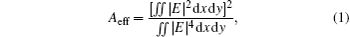where the double integral domain is the whole cross section, and E is transverse electric field distribution while THz radiation propagates.

Figure 2 shows the comparison of mode field area between the MIC and MMW hollow waveguide. From the figure, we can see clearly that mode field area varies linearly with the refractive index of measurand for the proposed MMW hollow waveguide, which is conducive to realizing the measurement of the measurand refractive index, while the mode field areas show irregular variations for the MIC hollow waveguide.

 Figure Option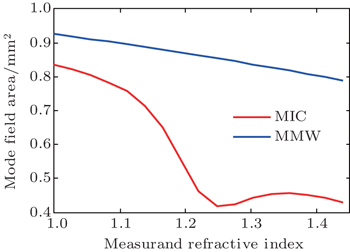Fig. 2. Variations of mode field area with measurand refractive index of MIC and MMW hollow waveguide.

Figure 3 indicates the mode field distributions of the proposed THz waveguide for 0.39 THz, 0.69 THz, and 0.99 THz frequencies, respectively. The refractive index of the measurand is assumed to 1.22. From Figs. 3(a)3(c), we can observe that this proposed THz waveguide structure can effectively restrict the THz pulse in the central air core region. The THz pulse propagating along one metal wire is referred to as a Sommerfeld wave. Thus, in this paper, the THz pulses which propagate along the surfaces of the multi-metal-wires as surface plasmon polaritons with a radial polarization can be regarded as a composite wave of Sommerfeld waves. And the composite wave shows a similar behavior to the higher order mode of transverse electromagnetic (TEM) mode. As a result, the maximum intensity is localized in each metal bound, rather than in the center of the area. Besides, due to the superior reflection performances of the copper wires, the multi-metal-wires serve as an efficient THz radiation reflector, leading to the zero intensity in the dielectric region. Furthermore, it can be seen that at the lower frequencies, THz plasmonic modes mainly transmit in the central air core, but in the higher frequencies, the THz light gathers in the vicinity of the metallic wire cladding. As a result, we select here the frequency with the modest mode field distribution as an operating frequency.

 Figure Option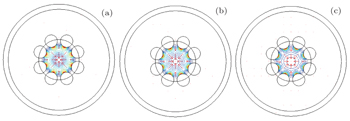Fig. 3. Mode field intensity distributions of the proposed THz waveguide at frequencies of (a) 0.39 THz, (b) 0.69 THz, and (c) 0.99 THz.

Figure 4 shows the variation of the modal effective refractive index with the variation of the measurand refractive index for the proposed THz waveguide. It is clearly seen that the modal effective refractive index of the THz waveguide changes obviously with measurand refractive index. Furthermore, modal effective index is defined as Re(β)/k0, where β is the propagation constant and k0 is the free space wave number. Just as in the conventional microwave hollow metallic waveguide where Re(β) < k0, when the measurand refractive index is 1, Re(β) values of the modes in the proposed THz waveguide are all smaller than k0, leading to modal effective index being less than 1. In addition, the modal effective refractive index varies linearly with the refractive index of measurand. According to the relation between the modal effective refractive index and the mode field area, we can find that this will be very helpful for measuring the measurand refractive index through the variation of the mode field area.

 Figure Option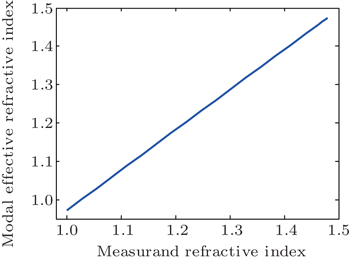Fig. 4. Variation of modal effective refractive index with measurand refractive index.

Next, we examine how the measurand refractive index influences mode field intensity distribution of the proposed THz waveguide. Figure 5 indicates the mode field distributions of the proposed THz waveguide for the measurand refractive indexes of 1.00, 1.22, and 1.44. Similarly, from these figures, it can be seen that for different measurands in the central core, this proposed THz waveguide structure can also effectively confine the plasmonic mode in the centeral air core region. Moreover, at the smaller measurand refractive index, THz plasmonic modes mainly transmit in the central air core, but at the larger measurand refractive index, the THz light gathers in the vicinity of the metallic wires cladding, and it can be found that the mode field area leads to a decreasing trend with the increase of refractive index of measurand. In what follows, we study numerically the variation of refractive index of measurand with MFA for THz waveguide.

 Figure Option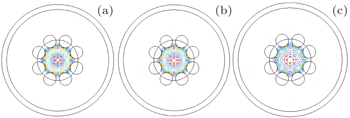Fig. 5. Mode field intensity distributions of the proposed THz waveguide at the refractive indexes of measurand of (a) 1.00, (b) 1.22, and (c) 1.44.

From the above discussion, we consider the relation between the mode field area and the refractive index of measurand. For different frequencies, variations of mode field area with the measurand refractive indexes are shown in Fig. 6. It can be seen that mode field area decreases with the increase of refractive index of measurand, which is consistent with the above conclusions. Meanwhile, for a specific measurand refractive index, the mode field area decreases with the increase of frequency, and the area change rate leads to a relatively great trend towards the larger frequency. In other words, the area change rate corresponds to the sensitivity of the THz waveguide sensor. For frequencies ranging from 0.39 THz to 0.99 THz, the sensitivities of the THz waveguide sensor are 0.137 mm2/RIU, 0.189 mm2/RIU, 0.237 mm2/RIU, 0.310 mm2/RIU, 0.409 mm2/RIU, 0.500 mm2/RIU, and 0.588 mm2/RIU, respectively. The sensitivity of the THz waveguide sensor gradually increases with the increase of the frequency. Moreover, from this figure, we can find that the mode field area variation curves appear to be smooth for the relatively low frequencies, while they appear to have some fluctuations for the relatively high frequencies. These fluctuations will interfere the measurement results of the measurand refractive indexes, and reduce the measurement accuracy. As a result, a trade-off between sensitivity and measurement accuracy must be made properly.

 Figure OptionFig. 6. Variations of mode field area with measurand refractive index for different frequencies.

In the above analyses, we only consider the case of fixed geometry dimensions. In the following, we will further analyze the variation relation between the mode field area and the measurand refractive index for different metallic wire diameters and air core diameters. Figure 7 shows the plots of mode field area versus measurand refractive index for different values of metallic wire diameter d2. In this figure, we can observe that the mode field area decreases generally with the increase of the measurand refractive index for each of corresponding metallic wire diameters. Moreover, for the fixed measurand refractive index, the mode field area decreases with the increase of metallic wire diameter except d2 = 0.48 mm. In addition, the fluctuation of mode field area appears in the higher measurand refractive index for several metallic wire diameters, which means that the measurement accuracy of measurand refractive index decreases to some extent, e.g., for d2 = 0.48 mm. In order to improve the measurement accuracy of measurand refractive index, we can select the THz waveguide with proper metallic wire diameter. Furthermore, it can be seen that the sensitivities are nearly the same for the different metallic wire diameters. Therefore, we can only consider the measurement accuracies here for different metallic wire diameters.

 Figure Option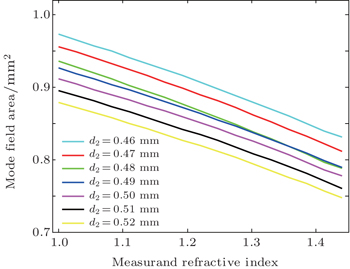Fig. 7. Variations of mode field area with measurand refractive index for different values of metallic wire diameter d2.

Figure 8 shows the plots of mode field area versus the measurand refractive index for different values of central air core diameter d1. From the figure, we can find that the variation of mode field area leads to a decreasing trend towards the higher measurand refractive index for each corresponding air core diameter d1. While for a fixed measurand refractive index, the mode field area curves exhibit irregular variations with the increasing of the air core diameter d1, but it does not affect the choice of appropriate THz waveguide dimensions to measure the refractive index of measurand. In the same way, fluctuation curve of the mode field area can affect the measurement accuracy, and the mode field area curves with fluctuation for an fixed air core diameter, such as the curves for d1 = 1.40 mm and d1 = 1.70 mm, should not be chosen and used when possible. Moreover, with the change of the air core diameter, the sensitivity of the THz waveguide sensor changes to a certain extent. For the air core diameters from 1.30 mm to 1.90 mm, the corresponding sensitivities of the THz waveguide sensor are 0.296 mm2/RIU, 0.383 mm2/RIU, 0.366 mm2/RIU, 0.310 mm2/RIU, 0.347 mm2/RIU, 0.310 mm2/RIU, and 0.303 mm2/RIU, respectively. In view of the measurement accuracy, the structure geometry with a higher sensitivity can be selected for measuring the the measurand refractive index.

 Figure Option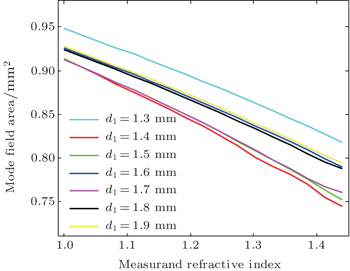Fig. 8. Plot of mode field area versus measurand refractive index for different values of air core diameter d1.

From the above analyses, we can find that the operating frequency has a greater effect on the sensitivity of the sensor, and the structure parameters have lesser effects on the sensitivity of the sensor. However, the structure parameters have greater effects on the measurement accuracy when the measurand refractive index is relatively large. As a result, considering the sensitivity and the measurement accuracy simultaneously, we select a THz waveguide structure with modest structure parameters and operating frequency, i.e., the air core diameter d1 = 1.50 mm, metallic wire diameter d2 = 0.49 mm, and operating frequency f = 0.89 THz. Figure 9 shows the fitting line of the mode field area versus the measurand refractive index for these parameters. From this figure, it can be observed that the mode field area has a linear dependence on the measurand refractive index, and the THz waveguide sensor has a higher measurement accuracy. The sensitivity of the THz waveguide sensor is about 0.585 mm2/RIU. From here we see that the reasonable choice of the structure parameters and operating frequency can optimize appropriately the measurement accuracy and sensitivity of the proposed THz waveguide refractive index sensor.

 Figure Option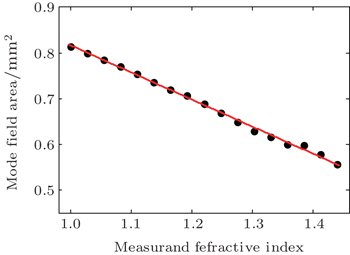Fig. 9. Mode field area as a function of measurand refractive index for d1 = 1.5 mm, d2 = 0.49 mm, and f = 0.89 THz.
4. Conclusions

In this paper, we propose and demonstrate a new kind of terahertz refractive index sensor based on multi-metal-wire hybrid-cladding hollow waveguide. Unlike the previously reported THz waveguide refractive index sensor, the proposed sensor measures the variation relation between the mode field area and the refractive index of the measurand. By the finite element method, the modal effective refractive index, mode field intensity distribution, and mode field area versus the measurand refractive index with different frequencies and waveguide structure dimensions are investigated. In addition, the variation of sensitivity with the geometric dimension of the device is also analyzed. Results show that the proposed THz refractive index sensor can achieve easily the measurement of the measurand refractive index, and the reasonable choices of the operating frequency and structure parameters can optimize appropriately the sensitivity and measurement accuracy. Through the demonstration of the measuand refractive index range, we can find that the proposed THz waveguide refractive index sensor is of potential application to detecting various gaseous measurands.

Reference
 1 Debus CBolivar P H 2007 Appl. Phys. Lett. 91 184102 2 Klatt GNagel MDekorsy TBartels A 2009 Electron. Lett. 45 310 3 Sizov F 2010 Opto-Electron. Rev. 18 10 4 Yao J Q Chi N Yang P F Cui H X Wang J L Li J S Xu D G Ding X 2009 Chin. J. Lasers 36 2213 (in Chinese) 5 Kleine-Ostmann TNagatsuma 2011 J. Infrared Milli. Terahz. Waves 32 143 6 Song H JAjito KMuramoto YWakatuski ANagatsuma TKukutsu N 2012 Electron. Lett. 48 953 7 Mittleman D MGupta MNeelamani RBaraniuk GRudd J VKoch M 1999 Appl. Phys. B 68 1085 8 Amanti M IScalari GBeck MFaist J 2012 Opt. Express 20 2772 9 Watts C MShrekenhamer DMontoya JLipworth GHunt JSleasman TKrishna SSmith D RPadila W J 2014 Nat. Photon. 8 605 10 Chen D RChen H B 2010 Opt. Express 18 3762 11 Yin G BLi S GWang X YLiu S 2011 Chin. Phys. B 20 090701 12 Xu D GWang Y YYu HLi J QLi Z XYan CZhang HLiu P XZhong KWang W PYao J Q 2014 Chin. Phys. B 23 054210 13 Mendis RGrischkowsky 2000 J. Appl. Phys. 88 4449 14 Chen L JChen H WKao T FLu J YSun C K 2006 Opt. Lett. 31 308 15 Lai C HHsueh Y CChen H WHuang Y JChang H CSun C K 2009 Opt. Lett. 34 3457 16 Wang K LMittleman D M 2004 Nature 432 376 17 McGowan R WGallot GGrischkowsky D 1999 Opt. Lett. 24 1431 18 Mendis RGrischkowsky D 2001 Opt. Lett. 26 846 19 Anthony JLeonhardt RArgyros A 2013 Opt. Express 21 2903 20 Sun BTang XZeng XShi Y 2012 Appl. Opt. 51 7276 21 Yu Y YLi X YSun BHe K P 2015 Chin. Phys. B 24 068702 22 Li J NShah C MWithayachumnankul WUng B S YMitchell ASriram SBhaskaran MChang S JAbbott D 2013 Appl. Phys. Lett. 102 121101 23 Kurt HCitrin D S 2005 Appl. Phys. Lett. 87 241119 24 Fan FChen SLin WMiao Y PChang S JLiu BWang X HLin L 2013 Appl. Phys. Lett. 103 161115 25 Fan FChen SWang X HWu P FChang S J 2015 IEEE Photon. Technol. Lett. 27 478 26 You BLu J YYu C PLiu T APeng J L 2012 Opt. Express 20 5858 27 Markov ASkorobogatiy M 2013 Appl. Phys. Lett. 103 181118 28 Nielsen KRasmussen H KJepsen P UBang O 2010 Opt. Lett. 35 2879 29 Harrington J AGeorge RPedersen PMueller E 2004 Opt. Express 12 5263 30 Cao Y Li R M Tong Z R 2013 Acta. Phys. Sin. 62 084215 (in Chinese)